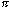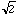Date: Thu, 17 Sep 1998 21:56:56 -0700 Subject: rational & irrational numbers I am trying to explain rational & irrational numbers to my niece who is grade 7 and am having difficulties. Could you please explain the difference between rational & irrational numbers. Thank you Ri Hi Ri Numbers that can be written in the form p/q where p and q are integers are known as rational numbers. Included in these are the integers (take q = 1). Thus for example the rationals include {0, 5/2, -18, -4/3, 27/5}. We unually write rational numbers in their lowest terms, for example 8/10 is usually written 4/5. We commonly write rationals in decimal form, so that 1/4 is the same as 0.25, 13/8 = 1.625 and 4/5 = 0.8. Some rationals, however, when written in decimal form don't stop a few places after the decimal. For example 1/3 = 0.333..., 10/11 = 0.909090... and 3/13 = 0.230769230769... When you write a rational number in decimal form you obtain either a decimal that stops after a finite number of terms, or a pattern that repeats as in the latter three examples.    Not all numbers are integers or rational numbers. If you construct a decimal that does not terminate and does not repeat it is not a rational number. For example 0.102003000400005... Numbers that are not rational are called irrational. The most famous of these is the ratio of the circumference of a circle to its diameter, called.is approximately 3.14159265358979323846; no matter how far we take the decimal expansion, it never repeats. Another irrational number iswhich is approximately 1.4142135623731.    Here is a way you can construct your own irrational number, or at least start because you will never finish. Start with 0 and then toss a coin. If you get a head write 0.1 and if you get a tail write 0.0. Now toss the coin again and write the second decimal place as a 1 if you tosses a head and 0 if you tosses a tail. So now you have either 0.10, 0.11, 0.00 or 0.01. Toss the coin again to get the third place after the decimal. Repeat forever.    In the days of Pythagoras (570-490 BC) the beauty of the rational numbers was revered. Pythagoras refused to accept the existence of the irrational numbers and it is said that one of his students, Hippasus, was sentenced to death for his refusal to deny their existence. Cheers, Penny

Go to Math Central

To return to the previous page use your browser's back button.